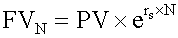Tags
Question

If the number of compounding periods becomes infinite, interest is compounding continuously. Accordingly, the future value N years from now is computed as follows:

[...]Tags
Question

If the number of compounding periods becomes infinite, interest is compounding continuously. Accordingly, the future value N years from now is computed as follows:

[...]
?

Tags
Question

If the number of compounding periods becomes infinite, interest is compounding continuously. Accordingly, the future value N years from now is computed as follows:

[...]If you want to change selection, open document below and click on "Move attachment"

subject 3. The Future Value and Present Value of a Single Cash Flow
t? The calculator keys to press are: Note that the answer is greater than when the compounding was annual. This is because interest is earned twice a year instead of only once. <span>If the number of compounding periods becomes infinite, interest is compounding continuously. Accordingly, the future value N years from now is computed as follows: <span><body><html>

#### Summary

status measured difficulty not learned 37% [default] 0

No repetitions